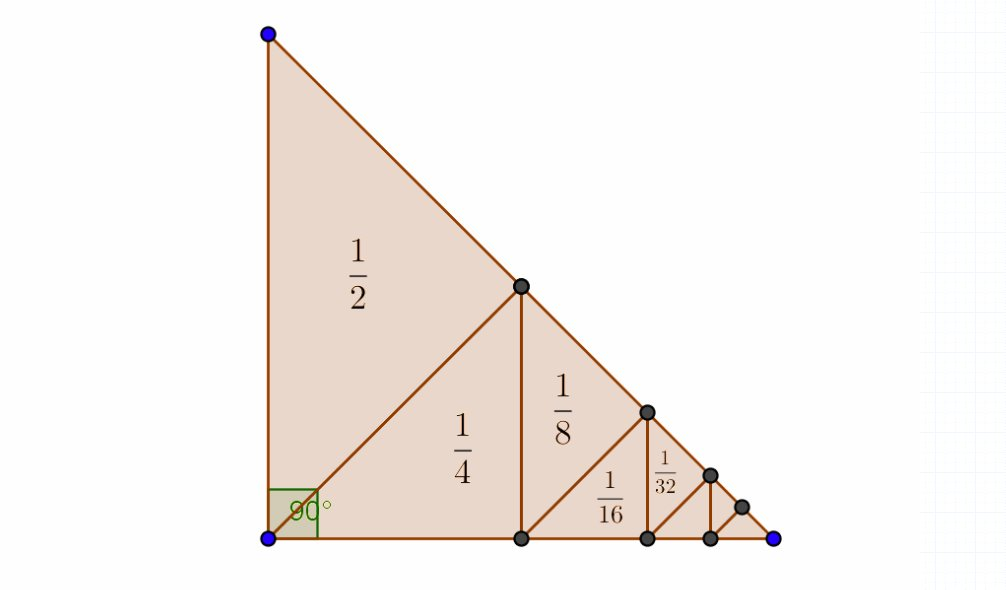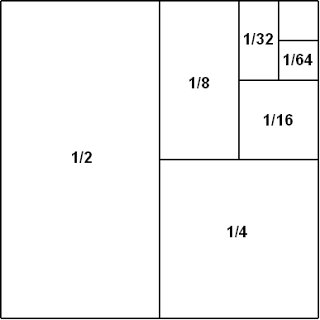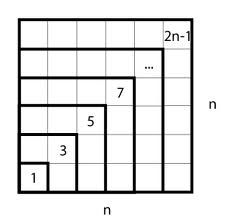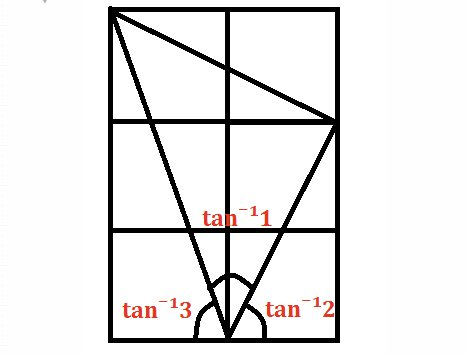# Proof Without Words #1

I decided to start a new series called ‘Proof Without Words’: a collection of pictures which prove a mathematical fact with an image. Remember, these are in no way rigorous and are just meant to give an idea of why the fact is true!

### 1/2+1/4+1/8+1/16+1/32+ … = 1### Sum of the first n positive odd numbers = n^2### arctan(1) + arctan(2) + arctan(3) = π### Viviani’s Theorem

The sum of the distances from any interior point to the sides of an equilateral triangle equals the length of the triangle’s altitude.M x

## 4 thoughts on “Proof Without Words #1”

1.howardat58 says:

These are lovely !

Like

1.mathsbyagirl says: